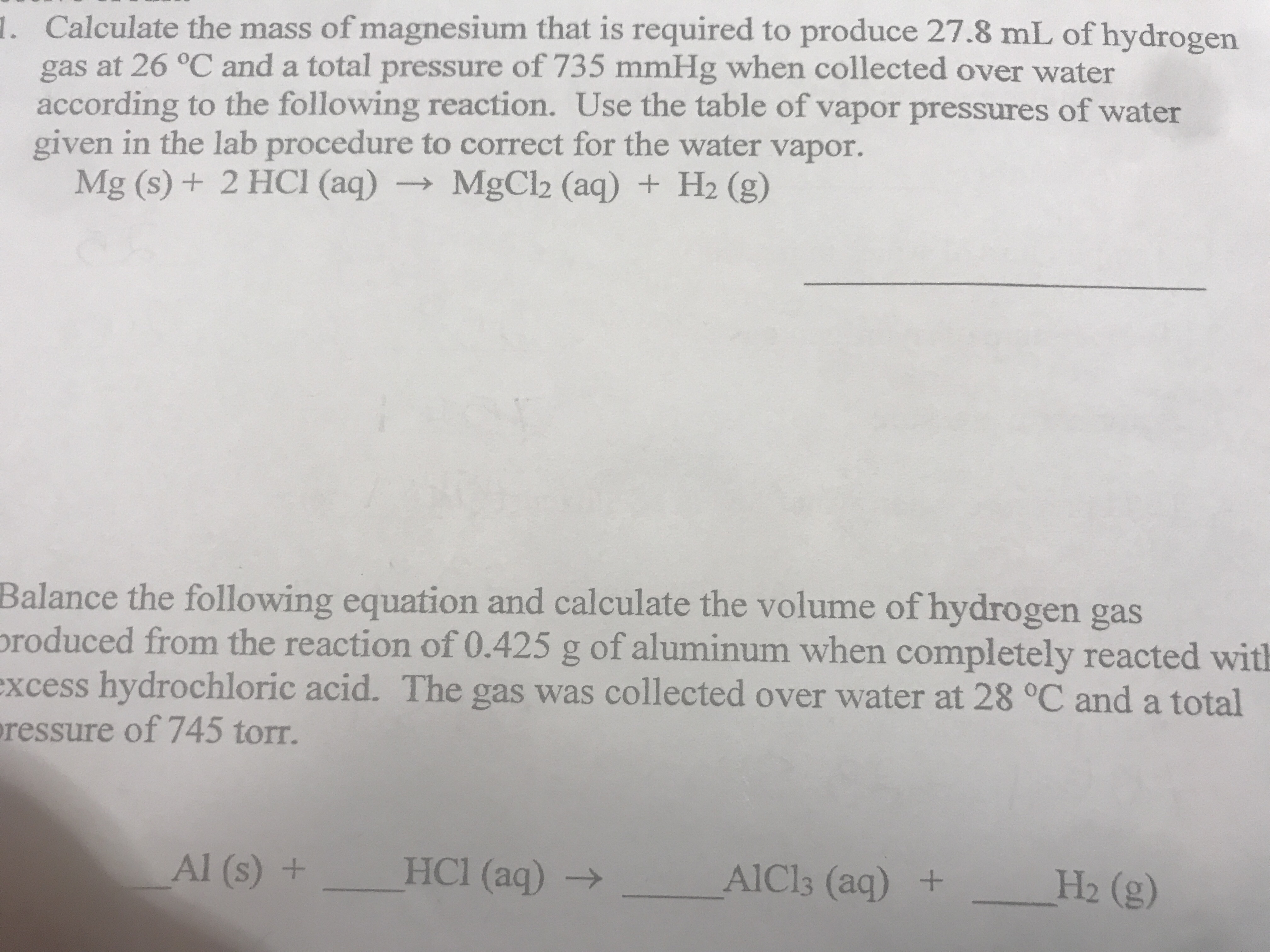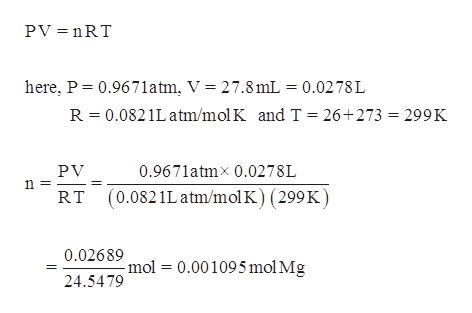# 1. Calculate the mass of magnesium that is required to produce 27.8 mL of hydrogengas at 26 °C and a total pressure of 735 mmHg when collected over wateraccording to the following reaction. Use the table of vapor pressures of watergiven in the lab procedure to correct for the water vapor.Mg (s) + 2 HCI (aq) MgCl2 (aq) + H2 (g)Balance the following equation and calculate the volume of hydrogen gasproduced from the reaction of 0.425 g of aluminum when completely reacted withexcess hydrochloric acid. The gas was collected over water at 28 °C and a totalressure of 745 torr.Al (s) +HCl (aq)AlCls (aq) +H2 (g)

Question
27 viewshelp_outlineImage Transcriptionclose1. Calculate the mass of magnesium that is required to produce 27.8 mL of hydrogen gas at 26 °C and a total pressure of 735 mmHg when collected over water according to the following reaction. Use the table of vapor pressures of water given in the lab procedure to correct for the water vapor. Mg (s) + 2 HCI (aq) MgCl2 (aq) + H2 (g) Balance the following equation and calculate the volume of hydrogen gas produced from the reaction of 0.425 g of aluminum when completely reacted with excess hydrochloric acid. The gas was collected over water at 28 °C and a total ressure of 745 torr. Al (s) + HCl (aq) AlCls (aq) + H2 (g) fullscreen
check_circle

Step 1

Given information,

Volume of hydrogen gas is 27.8 mL.

Temperature is 26 degree Celsius and pressure is 735 mmHg.

Step 2

Calculate the number of m...help_outlineImage TranscriptionclosePV nRT here, P 0.967 1atm, V = 27.8 mL = 0.0278 L R 0.0821Latm/mol K and T = 26+273 = 299K PV 0.9671atmx 0.0278L (0.0821Latm/mol K) (299K) RT 0.02689 -mol 0.001095 mol Mg 24.5479 fullscreen

### Want to see the full answer?

See Solution

#### Want to see this answer and more?

Solutions are written by subject experts who are available 24/7. Questions are typically answered within 1 hour.*

See Solution
*Response times may vary by subject and question.
Tagged in

### Gas laws